# Free Lunch (there is such a thing!)¶

Working with NYC Department of Education (DOE) to develop a method to optimize school lunch and breakfast reimbursements from the federal and state government.

## Overview¶

Providing free universal lunch to all students The National School Lunch Program, administered by the United States Department of Agriculture, subsidizes free or discounted lunch programs for children from low-income families in participating school districts. The New York City Department of Education is one of those districts. Traditionally, schools were reimbursed based on the number of meals served to eligible students. This caused concern, however, that this led to stigmatization of students based on the level of their family's income. As a result, advocates and officials have pushed for universal programs that provide free lunch to all students, regardless of income. In 2010, the Community Eligibility Provision (CEP) added to the National School Lunch Program to allow schools to receive an omnibus reimbursement based on the income demographics of their students, rather than a reimbursement for individual number of meals. Participating schools are required to provide breakfast and lunch at no charge to all students. After a pilot in the 2014-2015 school year, New York City announced its full participation in the program in September 2017.

MODA worked with the Office of School Support Services at the DOE to develop a Monte-Carlo method optimization algorithm to maximize the reimbursement it received from the federal government. The project also demonstrates how using open source software, such as Python, turned an intractable problem into one solvable on a single laptop.

## Reimbursement Rules:¶

Schools are reimbursed at either the "free" or "paid" rate for each meal. The fraction of meals which are reimbursed at the "free" rate, the higher of the two, is determined by the makeup of the group the school is enrolled under. This fraction we call the $threshold$. The rest of the meals get reimbursed at the "paid" rate.

At a minimum, the paid rate, $r_{lunch/breakfast,paid}$, for all meals is subsidized. This includes total number of breakfasts for all schools $\sum_{s}B_s$, and total number of lunches for all schools $\sum_{s}L_s$. That means the base reimbursement, $R_{base}$, is independent of the groupings:

\begin{align} R_{base} = r_{lunch,paid} \sum_{s}L_s + r_{breakfast,paid} \sum_{s}B_s \end{align}

So then total Reimbursement, $R$, is

\begin{align} R = \sum_{s} threshold_s (\Delta r_{lunch} L_s + \Delta r_{breakfast} B_s) + R_{base} \end{align}

where \begin{align} &\Delta r_{breakfast} = r_{breakfast,free} - r_{breakfast,paid} \\ &\Delta r_{lunch} = r_{lunch,free} - r_{lunch,paid} \end{align}

and the $threshold_s$ for each school, $s$, is determined it's group, $g$. It is the fraction of "identified students" $I_s$ for all schools in the group $g$ to the total number of "enrolled students" $N_s$ for all schools in the group, multiplied by a constant. This constant is set by the USDA at 1.6 but may change in subsequent years. $I_s$ and $N_s$ are based on enrollment in the previous school year. "Identified students" are those deemed categorically eligible for free meals, primarily due to receiving some sort of public assistance benefit.

\begin{align} threshold_s =Min(1, \frac{ \sum_{s\in g} I_s} {\sum_{s\in g} N_s } * 1.6 ) \end{align}

Before the school year begins, the groups are set and reported to the state. How schools should be grouped together is not prescribed.

Reimbursements to school districts happen on a monthly basis after the school year begins and are determined by the actual number of breakfasts and lunches eaten by the students. The groupings, however, must be submitted before the reimbursement, and so they can only be based on projected meal counts.

## Grouping Rules¶

• All groups have to meet the minimum threshold: $threshold_{min} = 40\% * 1.6 = 64\%$
• There can be at most 10 groups (this is not a hard rule, but an administrative limitation)
• All schools must be part of the program

## Objective¶

Find the optimum groupings to maximize reimbursement, $R$.

## Challenge¶

For any given grouping, it is relatively easy to calculate the reimbursement. The problem is that the number of possible groupings quickly grows out of control.

In combinatorics, the number of ways to group a set of n objects into k (non-empty) groups is called the Stirling partition number, denoted by $S(n,k)$. For instance there are 3 ways to partition 3 items into 2 groups.

\begin{align} &S(3,2)=3\\ &S(10,4) = 34,105\\ &S(30,4) = 4.8 * 10^{16}\\ &S(100,4) = 6.7 * 10^{58}\\ \end{align}

So with even just 100 schools and 4 groups brute forcing this calculation becomes impossible. To put it in perspective, even using the worlds fastest computers, assuming they can do quadrillions ($10^{15}$) of calculations per second, it would take more than the age of the universe to calculate reimbursements for 100 schools and all their possible groupings.

In :
import pandas as pd
import numpy as np
import time
import multiprocessing as mp
import matplotlib.pyplot as plt
%matplotlib inline
#plt.style.use('seaborn-poster')
#plt.style.use('fivethirtyeight')
import seaborn as sns
sns.set_context('poster',font_scale=1.2)

/usr/local/lib/python2.7/dist-packages/pandas/core/computation/__init__.py:18: UserWarning: The installed version of numexpr 2.4.3 is not supported in pandas and will be not be used
The minimum supported version is 2.4.6

ver=ver, min_ver=_MIN_NUMEXPR_VERSION), UserWarning)

In :
#constants
multiplier = 1.6

# combined Federal and NY State meal reimbursement rates
freeLunch = 3.2999
paidLunch = 0.4399
freeBreakfast = 2.1413
paidBreakfast = 0.2923

deltaLunchRate = freeLunch - paidLunch
deltaBreakfastRate = freeBreakfast - paidBreakfast

t_min = 0.40 * multiplier


## Test data from DOE¶

In :
# reading in data
doe = pd.read_excel('data/Test Data for CEP Grouping.xlsx',sheetname="School Data",header=2)
doe.rename(columns={'Identified Student Count':'Identified'},inplace=True)
print doe.shape

(1487, 5)

Out:
School Enrollment Identified Breakfast Lunch
0 1019 268.0 114.0 13744.040323 32119.415317
1 1025 188.0 121.0 2660.633468 8954.467200
2 1034 348.0 262.0 18359.910484 39773.741129
3 1060 679.0 293.0 7340.885081 19996.654634
4 1061 291.0 99.0 4320.555242 25602.863216

### Data Dictionary¶

• School - the school unique id
• Enrollment - the total number of students enrolled at the school
• Identified - the number of 'identified' students enrolled at the school. (should be less than Enrollment)
• Breakfast - the projected annual number of breakfasts served at the school.
• Lunch - the projected annual number of lunches served at the school

The Breakfast and Lunch columns are both pojected values by DOE. The actual numbers are not available until after the school year begins, after the groupings are already set.

### CEP dataframe¶

In :
#some schools are listed as 0 enrollment, this is an artifact of how schools are counted.
#keep only schools with >0 enrollment can be part of cep program.
cep = doe[doe.Enrollment>0].copy()
cep.reset_index(inplace=True)
print 'number of schools:',cep.shape
print 'number of students:',round(cep.Enrollment.sum())
print 'number of identified students', round(cep.Identified.sum())
print 'overall identified student percentage', cep.Identified.sum()/cep.Enrollment.sum()

number of schools: 1453
number of students: 726514.0
number of identified students 329902.0
overall identified student percentage 0.454089403209

In :
# define a few more columns that are useful.
# 'meal' is the max reimbursement of meals per year for each school.
# 'mealPerStudent' is the max per student.
# 'baseThreshold' is the threshold of the school if it is not grouped.
# 'group' will hold the integer name of the group the school belongs too. initally set a single group.

cep['meal'] = deltaLunchRate*cep['Lunch'] + deltaBreakfastRate*cep['Breakfast']
cep['paidMeal'] = paidLunch*cep['Lunch'] + paidBreakfast*cep['Breakfast']
cep['mealPerStudent'] = cep['meal']/cep['Enrollment']
cep['baseThreshold'] = cep['Identified']/cep['Enrollment']*multiplier
cep['group'] = 0


Out:
index School Enrollment Identified Breakfast Lunch meal paidMeal mealPerStudent baseThreshold group
0 0 1019 268.0 114.0 13744.040323 32119.415317 117274.258362 18146.713784 437.590516 0.680597 0
1 1 1025 188.0 121.0 2660.633468 8954.467200 30529.287474 4716.773284 162.389827 1.029787 0
2 2 1034 348.0 262.0 18359.910484 39773.741129 147700.374114 22863.070557 424.426362 1.204598 0
3 3 1060 679.0 293.0 7340.885081 19996.654634 70763.728769 10942.269083 104.217568 0.690427 0
4 4 1061 291.0 99.0 4320.555242 25602.863216 81212.895439 12525.597826 279.082115 0.544330 0

## Calculating Reimbursements based on school groupings¶

In :
# all meals in the CEP program are reimbursed at a minimum at the paid rate
baseReimburse = paidLunch*cep.Lunch.sum() + paidBreakfast*cep.Breakfast.sum()

# reimbursements over the base, this is the part that's dependant on groupings
def calcReimburse(cep,cost=0):
''' calculates and returns the total reimbursments (above base) for schools in a particlar grouping
Parameters:
cep: dataframe where each row is a school. cep columns include: Identified, Enrollment, meal, group
cost: cost = 0 (default) no cost to dropping schools from the program
cost = 1 sets a high penalty for letting a group go below the min threshold'''

group_cep = cep.groupby('group')
df = pd.DataFrame(index= group_cep.indices) #each row represents a group

df['threshold'] =  (group_cep['Identified'].sum()  / group_cep['Enrollment'].sum()) * multiplier
df['meal']      =  group_cep['meal'].sum()

# enforcing threshold rules:
df['applied_threshold'] = df['threshold']
df.loc[df['applied_threshold']  > 1, 'applied_threshold'] = 1
df.loc[df['applied_threshold']  < t_min,'applied_threshold'] = 0 - cost*10**6

df['reimbursed'] = df['applied_threshold'] * df['meal']

return df.reimbursed.sum()

def setThreshold(cep):
''' calculates the threshold for each school based on its group
cep columns: Enrollment, Identified'''
for i in set(cep.group):
df = cep[cep.group==i]
cep.loc[cep.group == i,'threshold'] = df['Identified'].sum() / float(df['Enrollment'].sum()) * multiplier
return 0

In :
print ' base reimbursement (all schools reimbursed at paid rate):',"{:e}".format(baseReimburse)

print '\n if all schools were reimbursed at 100% threshold (all at free rate)\n\
then this is the additional reimbursment:','{:e}'.format(cep.meal.sum())
cep['group']=0
print '\n if all schools are put into a single group, \
the additional reimbursement (over base):','{:e}'.format(calcReimburse(cep))

 base reimbursement (all schools reimbursed at paid rate): 3.767395e+07

if all schools were reimbursed at 100% threshold (all at free rate)
then this is the additional reimbursment: 2.434417e+08

if all schools are put into a single group, the additional reimbursement (over base): 1.768709e+08


## Finding Maximum Reimbursements¶

Monte Carlo Methods - 1) stochastic hill climbing and 2) simulated annealing

The Metropolis-Hastings Monte Carlo method is a well known approach used to simulate complex, multi-dimensional probabilities where direct sampling is not feasible. For our purposes we are not interested in generating a probability distribution, but in finding the maximum value (or the highest reimbursement). So we we use two related methods: stochastic hill climbing and simulated annealing.

We set up a random walk where each new step is only based on the previous step and not the steps before that, i.e. it's "memoryless". (This is also sometimes called a Markov Chain).

For our case a "step" is randomly choosing a school and moving it to another group. If the resulting step is "better" (has higher reimbursement) than the previous, then the step is accepted. If not, the step is rejected, meaning you throw it out and start back at the previous position. Many steps are then looped over until the result converges. This is stochastic hill climbing.

Simulated annealing (code below) is similar but is more permissive in keeping steps that would otherwise be rejected. The idea is that allowing some of these to go through may give more flexibility in finding a global maximum. This method is modeled on physical processes where slowly lowering the temperature allows for the system to find the lowest energy state. For this we invent a temperature parameter and lower it by some delta until we get close to 0 (at which point it is the same as stochastic hill climbing).

In practice the two methods yielded very similar results, but with simulated annealing getting there slightly faster.

1. Initialize: start in a random state, randomly group the schools, or with all schools in one group
2. Potential new step: choose a random school and change its group to another random group.
3. Compare: calculate the difference in reimbursements $\Delta R$
4. Accept/Reject Step:
• If the new reimbursement is greater than the old, $\Delta R >0$, then accept the new state
• If the new reimbursement is less than the old,$\Delta R<0$, then accept with probability $e^{\Delta R/T}$
• Otherwise reject.
5. Repeat from step 2 until the result converges, meaning all subsequent steps are rejected.
In :
def simulated_annealing(cep, randomstart=True, seed=None,
ngroupstart=1,ngroups=10, Tmax=1, deltaT=0.1):
'''simulated annealing procedure - finding optimal grouping
cep: schools dataframe
randomstart: if False start with groups already set in group column, otherwise
if True (default) start by randomly assigning groups
seed: seed for random generator
ngroupstart: integer number of groups in the random start
ngroups: number of groups
Tmax: max value for "temperature"
deltaT: change in "temperature" at each step

returns a list of reimbursements at each step and the dataframe with the final groupings
'''

startTime = time.time()
cep.reset_index(drop=True,inplace=True)
rows=cep.shape

# start by grouping schools randomly
if randomstart:
np.random.seed(seed)
cep.loc[:,'group'] = pd.Series(np.random.randint(0,ngroupstart,size=rows),
index=cep.index)

# store the results
old = calcReimburse(cep)
results=[old]

# mc loop
for T in np.arange(Tmax,0,-deltaT):
for i in range(1000):
df = cep.copy()

# choose a random school and move it to a different random group
df.loc[np.random.randint(0,rows),'group'] = np.random.randint(0,ngroups)

# calculate the reimbursement
new = calcReimburse(df,cost=1)
step = new - old

#keep move if reimbursement increases
if (step > 0):
old=new
cep.loc[:,'group'] = df.group
results.append(new)

#maybe keep move if reimbursement decreases, depending on how much
elif (np.random.uniform() < np.exp(step/T)):
old=new
cep.loc[:,'group'] = df.group
results.append(new)

cep = regroup(cep) #combining groups close by
final = calcReimburse(cep)
results.append(final)
print final
print 'time in h', (time.time()-startTime)/60.0/60.0
return results,cep

def regroup(cep):
'''if cep has multiple thresholds within one percent of each other, this
combines them'''
setThreshold(cep)
tlist = cep.groupby(cep.threshold.apply(lambda x: round(x,2))).groups.keys()
for i,t in enumerate(tlist):
cep.loc[cep.threshold.apply(lambda x:round(x,2))==t,'group']=i
setThreshold(cep)
return cep

def sa_ensemble(cep,trials=10,randomstart=True,ngroupstart=1,ngroups=10,Tmax=1,deltaT=.1):
'''run simulated annealing a number of times (trials) and choose the best
(highest reimbursement) as the final'''
pool = mp.Pool(processes=4) # parrallel over 4 cores
results = [pool.apply_async(simulated_annealing,
args=(cep,)) for x in range(trials)]
results = [p.get() for p in results]

reimb_ensemble = [results[i][-1] for i in range(trials)]
cep_ensemble = [results[i] for i in range(trials)]

max_reimb = max(reimb_ensemble)
max_index = reimb_ensemble.index(max_reimb)

return cep_ensemble[max_index]


## simulated annealing run and output¶

In :
reimb, cep = simulated_annealing(cep, Tmax=1,deltaT=.01)
setThreshold(cep)

187887754.945
time in h 0.159832963877

Out:
0

Plotting out the reimbursement result at each step allows us to see if the run converged.

In :
pd.Series(reimb).plot()

Out:
<matplotlib.axes._subplots.AxesSubplot at 0x7f3cb8e0ead0>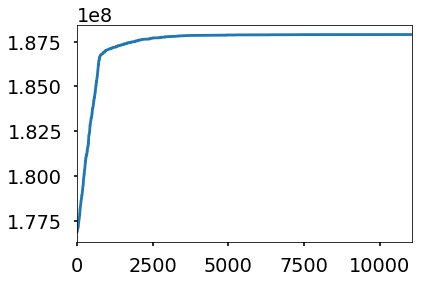baseThreshold is the threshold the school would have if it's in it's own group. Its' the percentage of identified students in the school multiplied by 1.6. The y-axis is the final threshold for the school after it's in it's optimized group.

In :
sns.pairplot(x_vars=['baseThreshold'],y_vars=['threshold'],data=cep, hue='threshold',
size=10,plot_kws={'s':100})

Out:
<seaborn.axisgrid.PairGrid at 0x7f3cb8602550>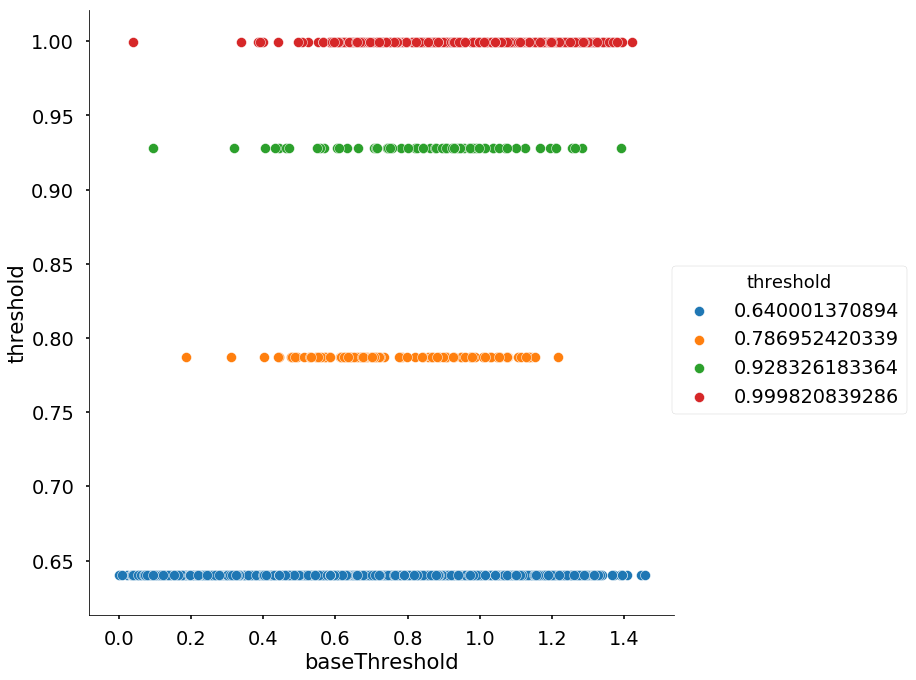In the graph below, each point is a school, the color represents its optimal group. The baseThreshold is the school's percent identified students times the multiplier 1.6. The mealPerStudent is the average reimbursement of the meal if it received full reimbursement (minus the lowest reimbursement cost).

In :
sns.pairplot(x_vars=['baseThreshold'],y_vars=['mealPerStudent'],data=cep, hue='threshold',
size=10,plot_kws={'s':100})

Out:
<seaborn.axisgrid.PairGrid at 0x7f3cb3c04c90>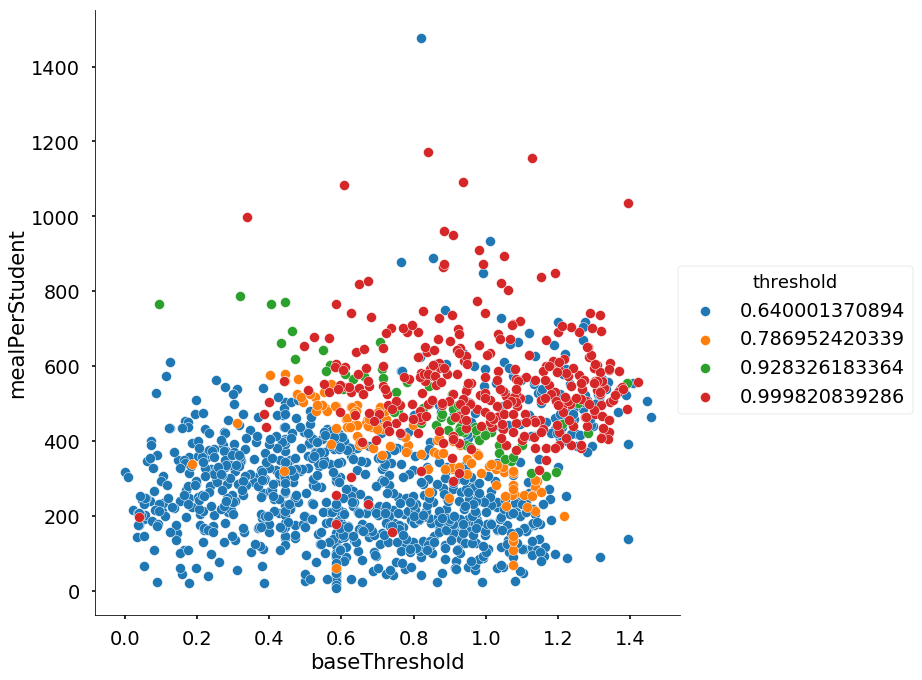In :
pd.concat([cep.groupby('threshold').count()[['School']],
cep.groupby('threshold').sum()[['Enrollment']],
cep.groupby('threshold').mean()[['mealPerStudent']]],axis=1)

Out:
School Enrollment mealPerStudent
threshold
0.640001 948 521650.323920 277.001756
0.786952 114 40981.906091 379.228622
0.928326 67 29602.747619 493.644167
0.999821 324 134278.988372 555.030739

## ensemble¶

Can we do any better? This is a stochastic method, meaning each time you restart you will get a slightly different answer. We can do this many times, utilizing python's parrallel processing capabilities, and choose the best of the lot.

In :
%%capture
cep = sa_ensemble(cep,trials=100,Tmax=1,deltaT=.01)

In :
calcReimburse(cep)

Out:
188209638.71381176
In :
sns.pairplot(x_vars=['baseThreshold'],y_vars=['threshold'],data=cep, hue='threshold',
size=10,plot_kws={'s':100})

Out:
<seaborn.axisgrid.PairGrid at 0x7f3cb8160650>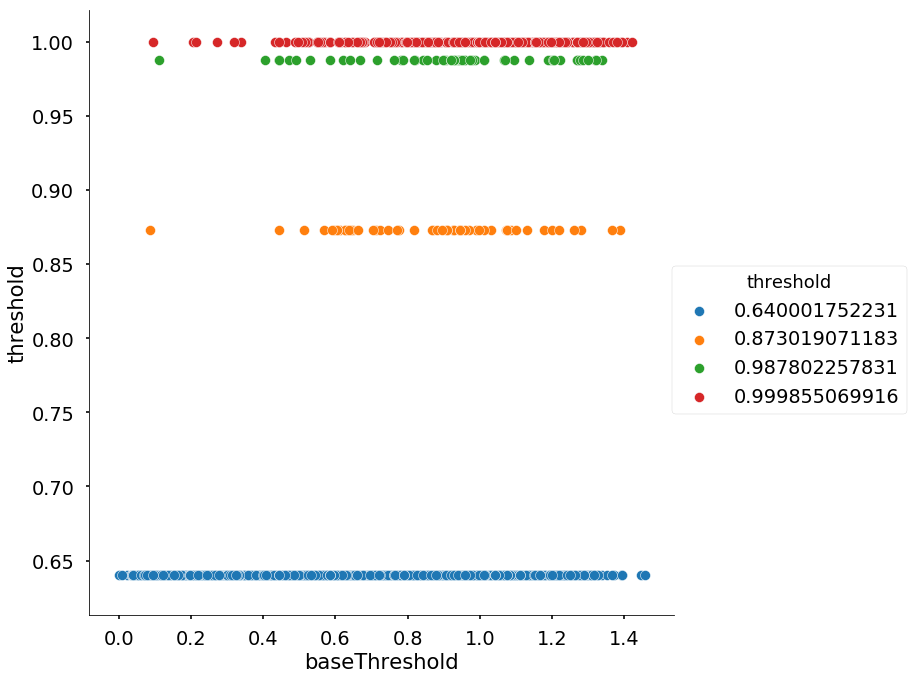In :
sns.pairplot(x_vars=['baseThreshold'],y_vars=['mealPerStudent'],data=cep, hue='threshold',
size=10,plot_kws={'s':100})

Out:
<seaborn.axisgrid.PairGrid at 0x7f3cb82599d0>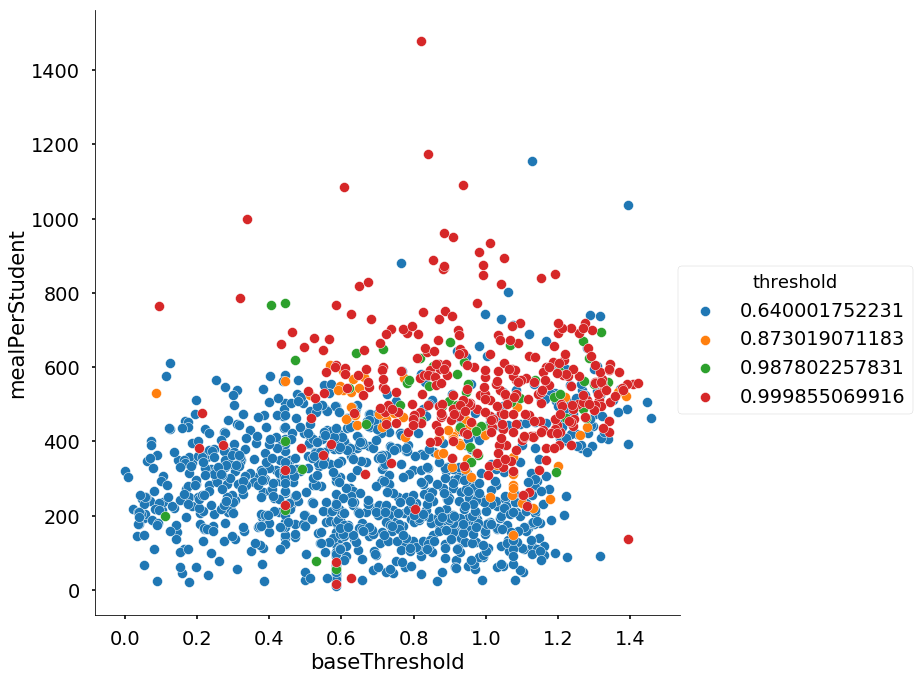In :
pd.concat([cep.groupby('threshold').count()[['School']],
cep.groupby('threshold').sum()[['Enrollment']],
cep.groupby('threshold').mean()[['mealPerStudent']]],axis=1)

Out:
School Enrollment mealPerStudent
threshold
0.640002 1013 541017.119823 283.998775
0.873019 61 28360.914286 425.450919
0.987802 59 23315.114950 496.070851
0.999855 320 133820.816944 549.445001
In :
mcReimburse =  baseReimburse+calcReimburse(cep)
cep1group=cep.copy()
cep1group.group = 0 # putting all schools into one group
oneGrpReimburse =  baseReimburse+calcReimburse(cep1group)
print '$reimbursement using MC technique:','{:.2e}'.format(mcReimburse) print '$ reimbursement all schools into a single group:','{:.2e}'.format(oneGrpReimburse)
print '$difference:','{:.2e}'.format(mcReimburse-oneGrpReimburse) print 'percent difference:','{:.2f}'.format(100*(mcReimburse-oneGrpReimburse)/oneGrpReimburse)  $ reimbursement using MC technique: 2.26e+08
$reimbursement all schools into a single group: 2.15e+08$ difference: 1.13e+07
percent difference: 5.29

In [ ]:


In [ ]:


In [ ]:


In [ ]: